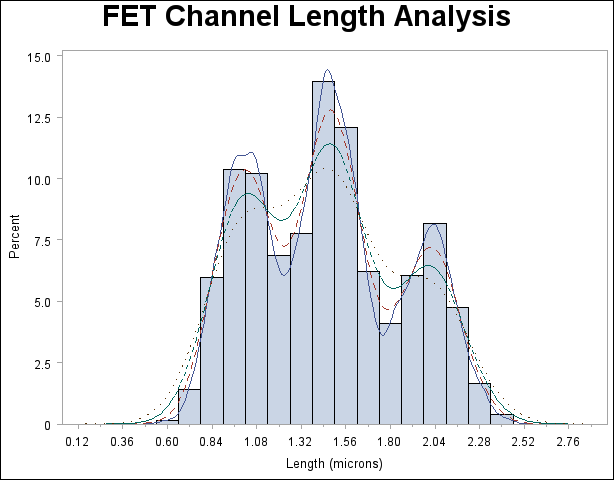The UNIVARIATE Procedure

## Example 4.23 Computing Kernel Density Estimates

This example illustrates the use of kernel density estimates to visualize a nonnormal data distribution. This example uses the data set Channel, which is introduced in Example 4.15.

When you compute kernel density estimates, you should try several choices for the bandwidth parameterbecause this determines the smoothness and closeness of the fit. You can specify a list of up to five C= values with the KERNEL option to request multiple density estimates, as shown in the following statements:

```title 'FET Channel Length Analysis';
proc univariate data=Channel noprint;
histogram Length / kernel(c = 0.25 0.50 0.75 1.00
l = 1 20 2 34
noprint);
run;
```

The L= secondary option specifies distinct line types for the curves (the L= values are paired with the C= values in the order listed). Output 4.23.1 demonstrates the effect of. In general, larger values ofyield smoother density estimates, and smaller values yield estimates that more closely fit the data distribution.

Output 4.23.1 Multiple Kernel Density EstimatesOutput 4.23.1 reveals strong trimodality in the data, which is displayed with comparative histograms in Example 4.15.

A sample program for this example, uniex09.sas, is available in the SAS Sample Library for Base SAS software.Previous Page | Next Page | Top of Page